# Term Answer Description Risk A. The risk of an asset when it is the only asset...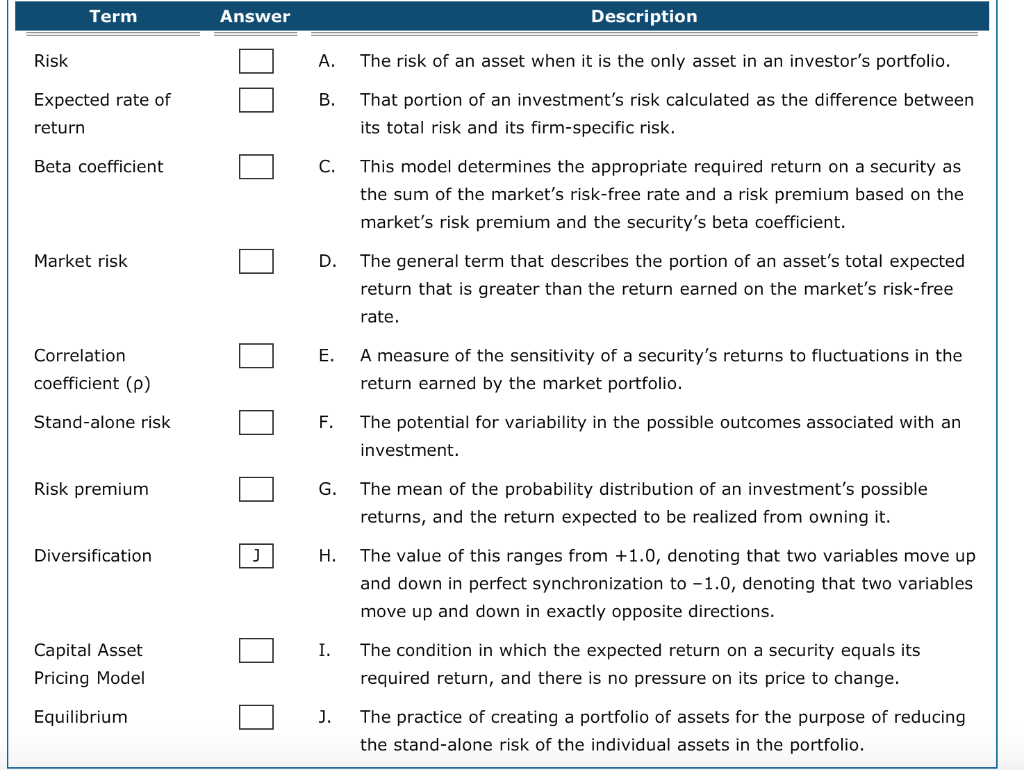Term Answer Description Risk A. The risk of an asset when it is the only asset in an investor's portfolio. Expected rate of return That portion of an investment's risk calculated as the difference between its total risk and its firm-specific risk. Beta coefficient This model determines the appropriate required return on a security as the sum of the market's risk-free rate and a risk premium based on the market's risk premium and the security's beta coefficient. Market risk The general term that describes the portion of an asset's total expected return that is greater than the return earned on the market's risk-free rate. E. Correlation coefficient (e) A measure of the sensitivity of a security's returns to fluctuations in the return earned by the market portfolio. Stand-alone risk F. The potential for variability in the possible outcomes associated with an investment. Risk premium O G. The me The mean of the probability distribution of an investment's possible returns, and the return expected to be realized from owning it. Diversification 0 H. The value of this ranges The value of this ranges from +1.0, denoting that two variables move up and down in perfect synchronization to -1.0, denoting that two variables move up and down in exactly opposite directions. The condition in which the expected return on a security equals its required return, and there is no pressure on its price to change. I. Capital Asset Pricing Model Equilibrium The practice of creating a portfolio of assets for the purpose of reducing the stand-alone risk of the individual assets in the portfolio.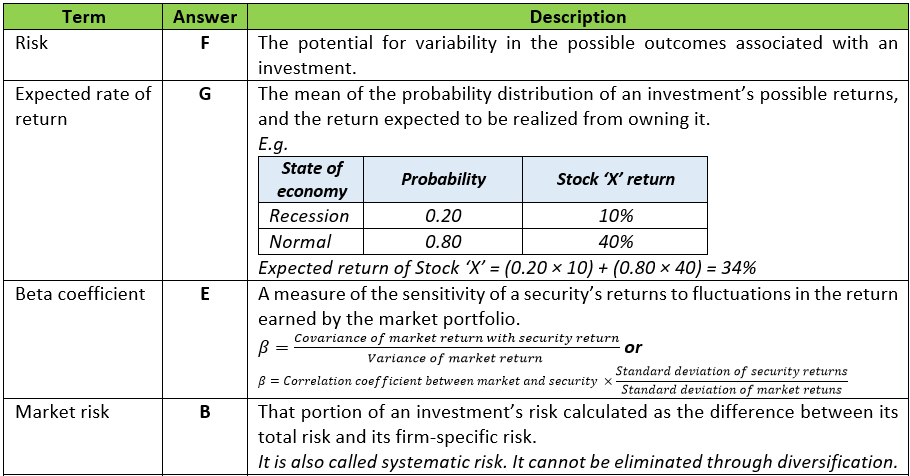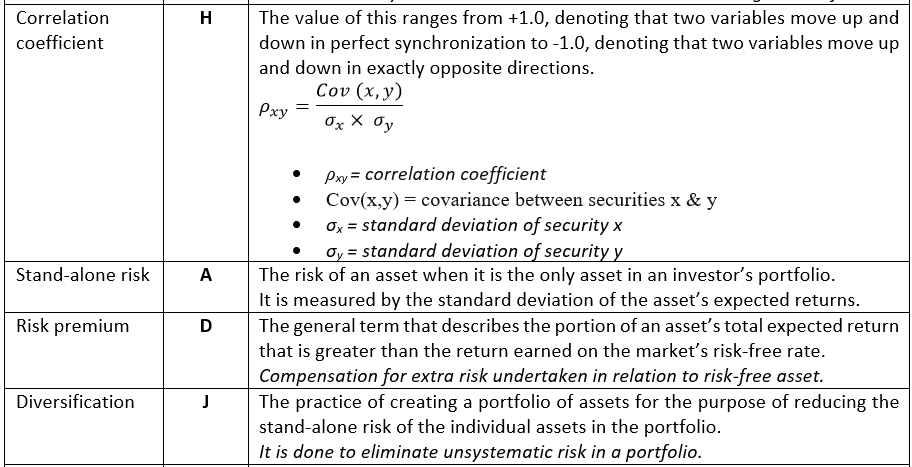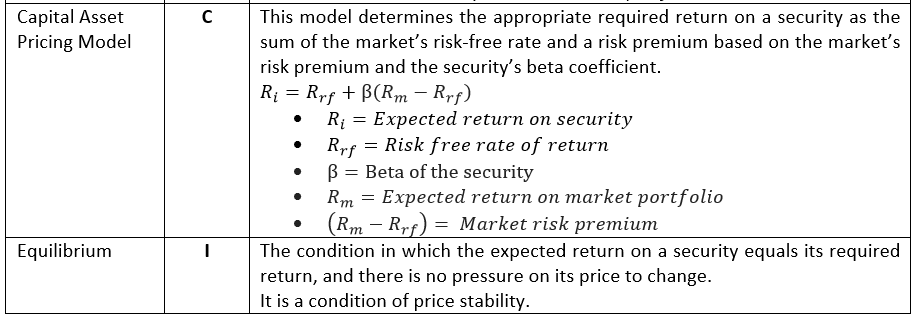#### Earn Coin

Coins can be redeemed for fabulous gifts.

Similar Homework Help Questions
• ### Term Answer Description Risk A. The potential for variability in the possible outcomes associated with an...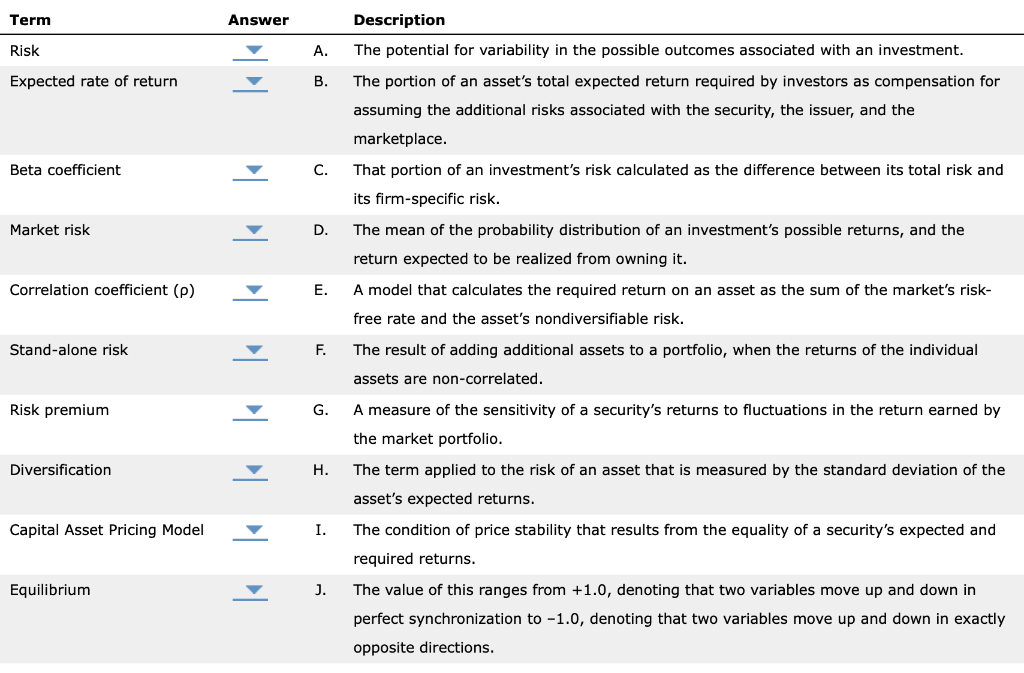Term Answer Description Risk A. The potential for variability in the possible outcomes associated with an investment. Expected rate of return The portion of an asset's total expected return required by investors as compensation for assuming the additional risks associated with the security, the issuer, and the marketplace. Beta coefficient That portion of an investment's risk calculated as the difference between its total risk and its firm-specific risk. Market risk The mean of the probability distribution of an investment's possible...

• ### Term Description Risk A. The term applied to the risk of an asset that is measured...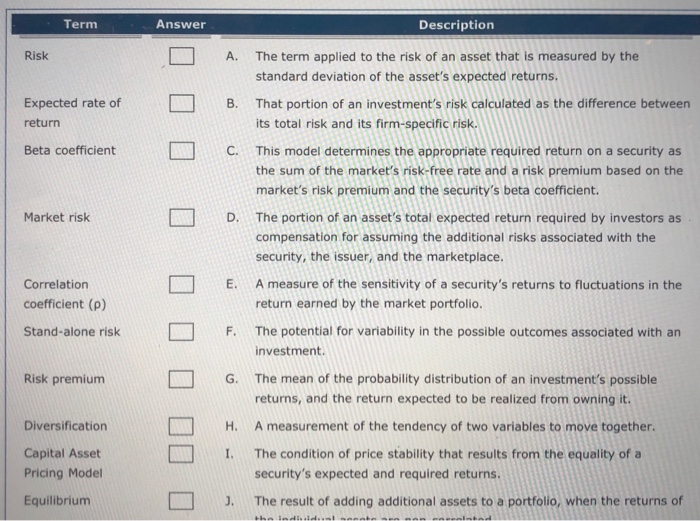Term Description Risk A. The term applied to the risk of an asset that is measured by the standard deviation of the asset's expected returns. That portion of an investment's risk calculated as the difference between its total risk and its firm-specific risk. Expected rate of return B. Beta coefficient L C . This model determines the appropriate required return on a security as the sum of the market's risk-free rate and a risk premium based on the market's risk...

• ### Ch 02: Assignment - Risk and Return: Part 1 Term Answer Risk A Expected rate of...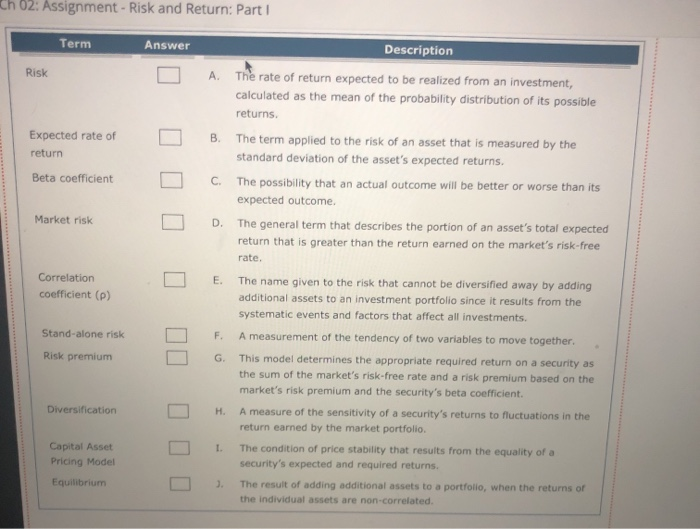Ch 02: Assignment - Risk and Return: Part 1 Term Answer Risk A Expected rate of return B Description The rate of return expected to be realized from an investment, calculated as the mean of the probability distribution of its possible returns. The term applied to the risk of an asset that is measured by the standard deviation of the asset's expected returns. The possibility that an actual outcome will be better or worse than its expected outcome The general...

• ### Term Answer Description A. The general term that describes the portion of an asset's total expected...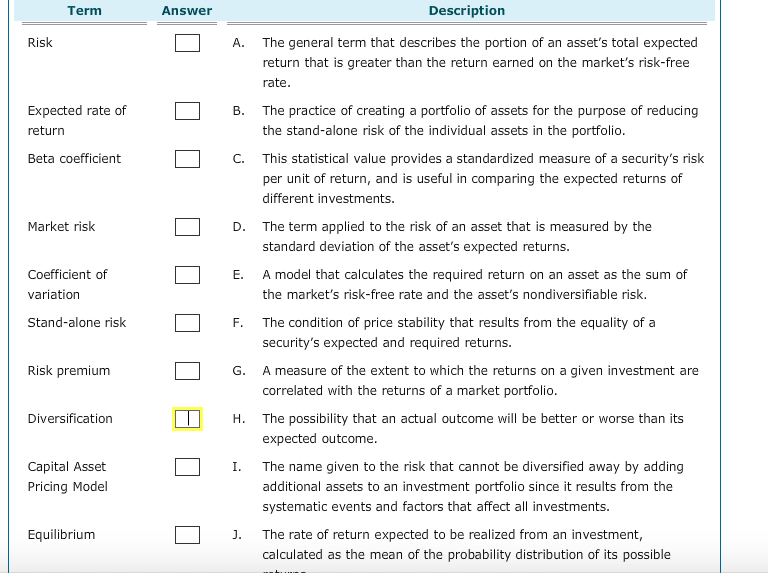Term Answer Description A. The general term that describes the portion of an asset's total expected return that is greater than the return earned on the market's risk-free rate Expected rate of return B. The practice of creating a portfolio of assets for the purpose of reducing the stand-alone risk of the individual assets in the portfolio Beta coefficient C. This statistical value provides a standardized measure of a security's risk per unit of return, and is useful in comparing...

• ### od The capital asset pricing model (CAPM) explains how risk should be considered when stocks and...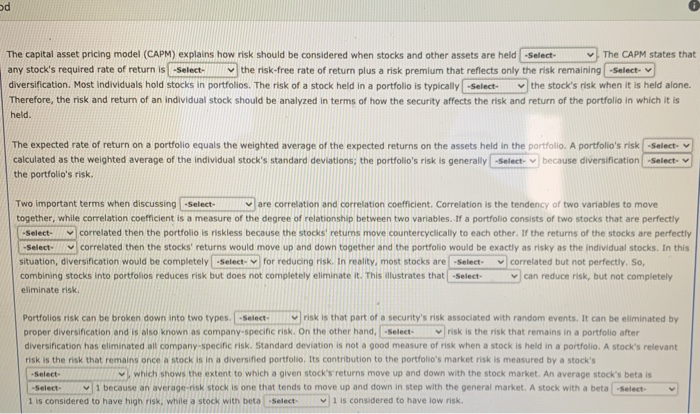od The capital asset pricing model (CAPM) explains how risk should be considered when stocks and other assets are held -Select- The CAPM states that any stock's required rate of return is -Select the risk-free rate of return plus a risk premium that reflects only the risk remaining -Select- diversification. Most individuals hold stocks in portfolios. The risk of a stock held in a portfolio is typically -Select the stock's risk when it is held alone. Therefore, the risk and...

• ### 2. 3: Risk and Rates of Return: Risk in Portfolio Context Risk and Rates of Return:...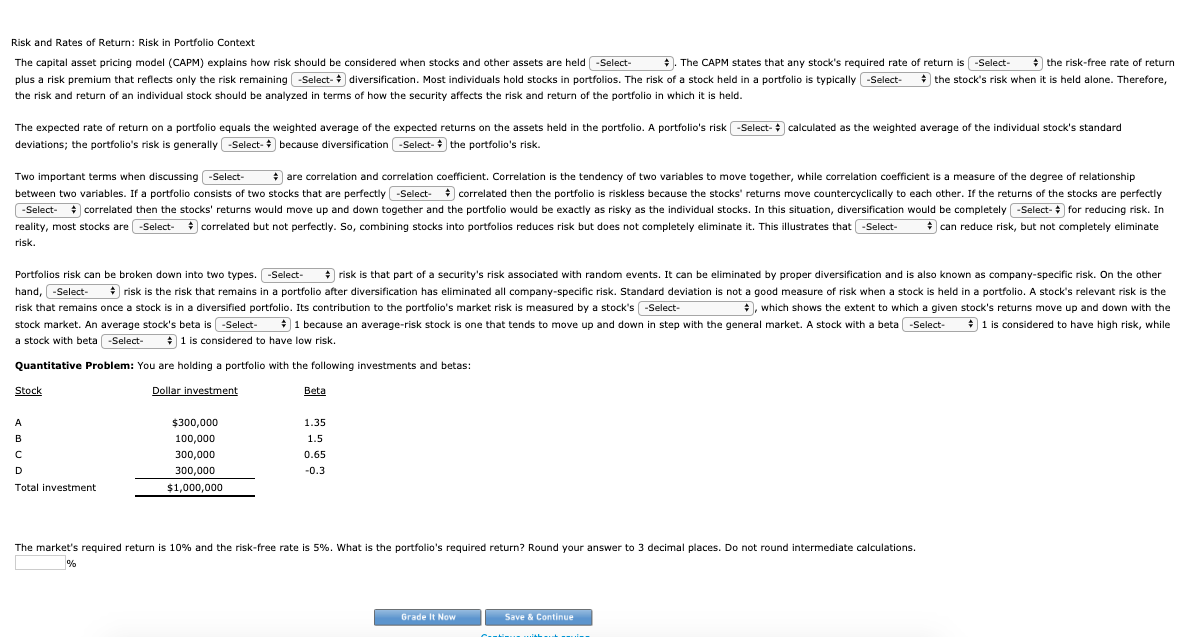2. 3: Risk and Rates of Return: Risk in Portfolio Context Risk and Rates of Return: Risk in Portfolio Context The capital asset pricing model (CAPM) explains how risk should be considered when stocks and other assets are held . The CAPM states that any stock's required rate of return is the risk-free rate of return plus a risk premium that reflects only the risk remaining diversification. Most individuals hold stocks in portfolios. The risk of a stock held in...

• ### The scroll down options are 1. systematic/unsystematic risk 2. systematic/unsystematic risk 3. standard deviation/risk aversion 4....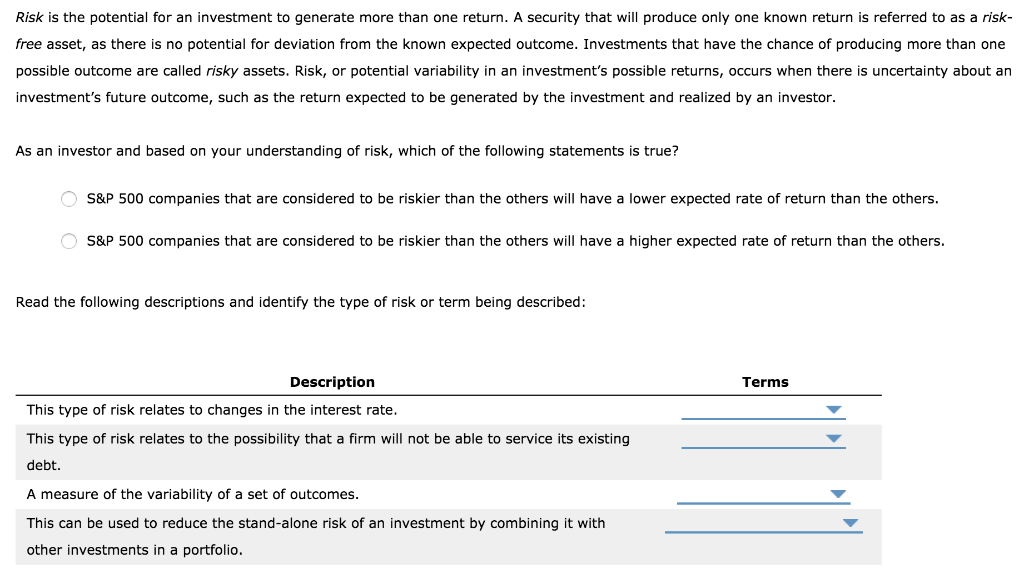The scroll down options are 1. systematic/unsystematic risk 2. systematic/unsystematic risk 3. standard deviation/risk aversion 4. correlation coefficient/diversification Risk is the potential for an investment to generate more than one return. A security that will produce only one known return is referred to as a risk- free asset, as there is no potential for deviation from the known expected outcome. Investments that have the chance of producing more than one possible outcome are called risky assets. Risk, or potential variability...

• ### Explain your answer in depth - show formulas and all relevant stuff The current market price of a security is \$50, the security's expected return is 15%, the riskless rate of interest is 2%, and...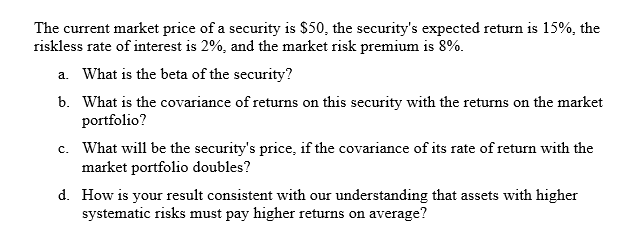Explain your answer in depth - show formulas and all relevant stuff The current market price of a security is \$50, the security's expected return is 15%, the riskless rate of interest is 2%, and the market risk premium is 8% What is the beta of the security? What is the covariance of returns on this portfolio? What will be the security's price, if the covariance of its rate of return with the market portfolio doubles? How is your result...

• ### In the following table, indicate whether each statement refers to the Capital Market Line (CML) or...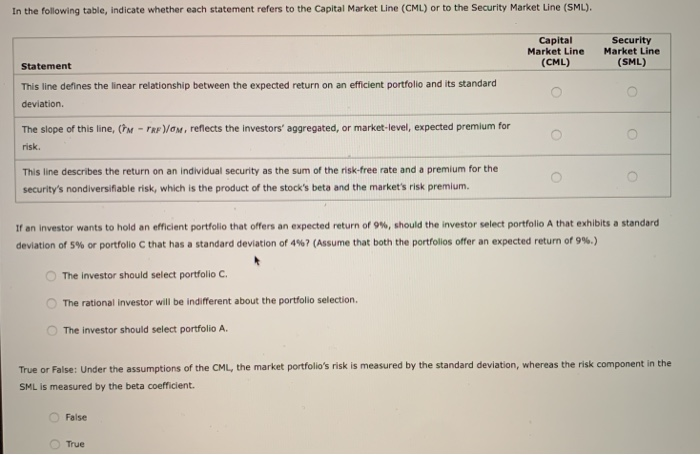In the following table, indicate whether each statement refers to the Capital Market Line (CML) or to the Security Market Line (SML). Capital Market Line (CML) Security Market Line (SML) Statement This line defines the linear relationship between the expected return on an efficient portfolio and its standard deviation The slope of this line, th risk. - TRP/OM, reflects the investors' aggregated, or market-level, expected premium for This line describes the return on an individual security as the sum of...

• ### Risk, value of systematic risk, risk free rate of return and market risk premium

Security A = return of 15%, SD of 8%, beta of 1.2Security B = return of 12%, SD of 14%, beta of 0.90a. Which of A and B has the least total risk? The least systematic risk?b. What is the value of systematic risk for a portfolio with 75% of the funds invested in A and 25% of the funds invested in B?c. Calculate the risk free rate of return and the market risk premium (i.e., Rf and RM –...